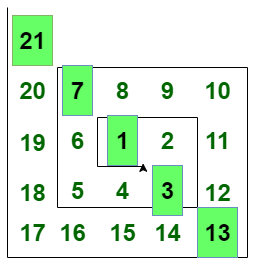# Find the Nth Hogben Numbers

Given a number N, the task is to print the Nth Hogben number.

Hogben Number: In a spiral arrangement of the integers, Hogben Numbers appear on the main diagonal (see the picture below).The first few Hogben numbers are 1, 3, 7, 13, 21, 31, 43, 57, 73, 91, 111, 133, 157, 183, 211, 241, 273……. and many more.

Example:

Input: N = 4
Output: 3
Explanation:
The 4th Hogben number that lies on the diagonal of the spiral pattern is 13.

Input: N = 7
Output: 43
Explanation:
The 7th Hogben number that lies on the diagonal of the spiral pattern is 43.

## Recommended: Please try your approach on {IDE} first, before moving on to the solution.

Approach:
We can observe from the sequence of Hogben numbers, that the Nth Hogben number HN is equal to.

Below is the implementation of the above approach.

## C++

 `// C++ program to print ` `// N-th Hogben Number ` ` `  `#include ` `using` `namespace` `std; ` ` `  `// Function returns N-th ` `// Hogben Number ` `int` `HogbenNumber(``int` `a) ` `{ ` `    ``int` `p = (``pow``(a, 2) - a + 1); ` `    ``return` `p; ` `} ` ` `  `// Driver code ` `int` `main() ` `{ ` `    ``int` `N = 10; ` ` `  `    ``cout << HogbenNumber(N); ` ` `  `    ``return` `0; ` `} `

## Java

 `// Java program to print ` `// N-th Hogben Number ` `import` `java.util.*; ` ` `  `class` `GFG{ ` `     `  `// Function returns N-th ` `// Hogben Number ` `public` `static` `int` `HogbenNumber(``int` `a) ` `{ ` `    ``int` `p = (``int``)(Math.pow(a, ``2``) - a + ``1``); ` `    ``return` `p; ` `} ` ` `  `// Driver code ` `public` `static` `void` `main(String args[]) ` `{ ` `    ``int` `N = ``10``; ` ` `  `    ``System.out.print(HogbenNumber(N)); ` `} ` `} ` ` `  `// This code is contributed by Akanksha_Rai `

## Python3

 `# Python3 program to print ` `# N-th Hogben Number ` ` `  `# Function returns N-th ` `# Hogben Number ` `def` `HogbenNumber(a): ` `     `  `    ``p ``=` `(``pow``(a, ``2``) ``-` `a ``+` `1``) ` `    ``return` `p ` ` `  `# Driver code ` `N ``=` `10` ` `  `print``(HogbenNumber(N)) ` ` `  `# This code is contributed by shubhamsingh10 `

## C#

 `// C# program to print ` `// N-th Hogben Number ` `using` `System; ` `class` `GFG{ ` `     `  `// Function returns N-th ` `// Hogben Number ` `public` `static` `int` `HogbenNumber(``int` `a) ` `{ ` `    ``int` `p = (``int``)(Math.Pow(a, 2) - a + 1); ` `    ``return` `p; ` `} ` ` `  `// Driver code ` `public` `static` `void` `Main() ` `{ ` `    ``int` `N = 10; ` ` `  `    ``Console.Write(HogbenNumber(N)); ` `} ` `} ` ` `  `// This code is contributed by Code_Mech `

Output:

```91
```

Don’t stop now and take your learning to the next level. Learn all the important concepts of Data Structures and Algorithms with the help of the most trusted course: DSA Self Paced. Become industry ready at a student-friendly price.

My Personal Notes arrow_drop_upIf you like GeeksforGeeks and would like to contribute, you can also write an article using contribute.geeksforgeeks.org or mail your article to contribute@geeksforgeeks.org. See your article appearing on the GeeksforGeeks main page and help other Geeks.

Please Improve this article if you find anything incorrect by clicking on the "Improve Article" button below.

1

Please write to us at contribute@geeksforgeeks.org to report any issue with the above content.